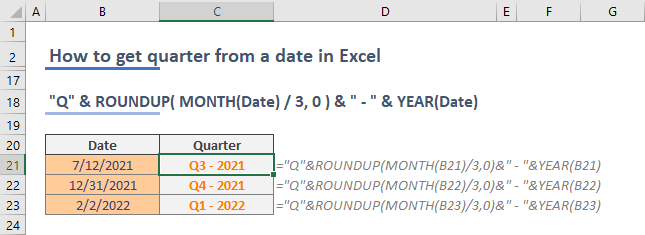In this guide, we're going to show you how to get quarter from a date in Excel.

To get quarter from a date, you simply need to divide the month by 3 and round up the result to the nearest integer.

=ROUNDUP( MONTH(Date) / 3, 0 )

Since each quarter of the year consists of 3 months, dividing the month of a date by 3 returns the number of 3-month intervals. For example, 6 months is two quarters, meaning that the month is in the 2nd quarter. If a number with decimal point is returns=ed, the whole part of the number indicates the previous quarter. For example, 7 by 3 equals to 2.33 approximately which means its in the 3rd quarter.

You can get the month number of a date easily by the MONTH function. The ROUNDUP function can round the given number up to the specific decimal digit. Since we need a whole number, the second argument of the ROUNDUP function should be 0.You can also add labels and even the year of the date to the quarter value.

=”Q” & ROUNDUP( MONTH(Date) / 3, 0 )If your Excel model covers multiple years, you can easily add the year of the evaluated date in a couple of ways:

1. The YEAR function returns the year from a given date just like in MONTH
2. Alternatively, you can use the TEXT function to get the year.

Prefer using the YEAR function to return a 4-digit year number.

"Q" & ROUNDUP( MONTH(Date) / 3, 0 ) & " - " & YEAR(Date)Check out the TEXT function and number formatting in Excel to modify any date without extensive formulations. To get a 2-digit year notation, use the TEXT function with “YY” format.

"Q" & ROUNDUP( MONTH(Date) / 3, 0 ) & "'" & TEXT(Date, "YY" )